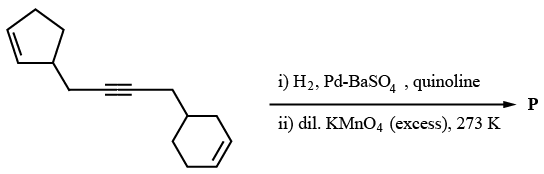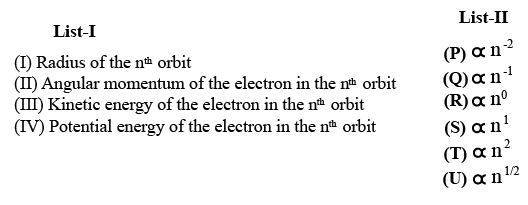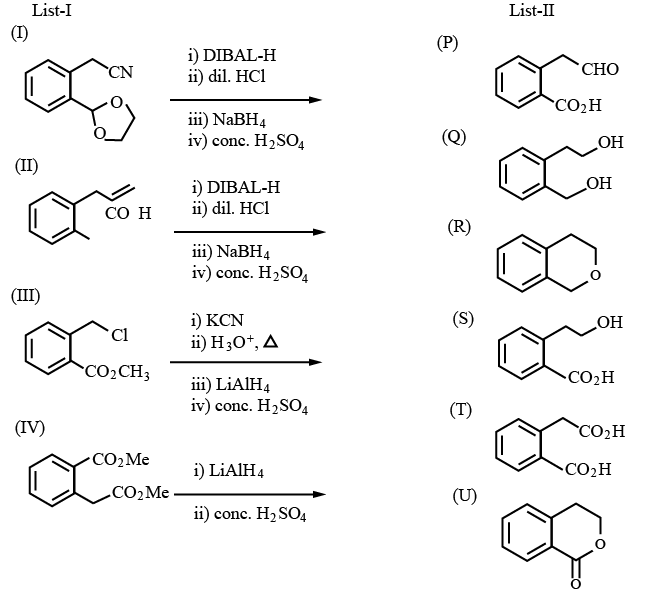Instructions

For the following questions answer them individually

Question 31

# Total number of hydroxyl groups present in a molecule of the major product P is ______ .Backspace
789
456
123
0.-
Clear AllQuestion 32

# Total number of isomers, considering both structural and stereoisomers, of cyclic ethers with the molecular formula $$C_4H_8O$$ is

Backspace
789
456
123
0.-
Clear AllInstructions

Answer by appropriately matching the lists based on the information given in the paragraph
Consider the Bohr’s model of a one-electron atom where the electron moves around the nucleus. In the following, List-I contains some quantities for the $$n^{th}$$ orbit of the atom and List-II contains options showing how they depend on n.Question 33

# Which of the following options has the correct combination considering List-I and List-II?Question 34

# Which of the following options has the correct combination considering List-I and List-II?Instructions

Answer by appropriately matching the lists based on the information given in the paragraph
List-I includes starting materials and reagents of selected chemical reactions. List-II gives structures of compounds that may be formed as intermediate products and/or final products from the reactions of List-I.Question 35

# Which of the following options has correct combination considering List-I and List-II?Question 36

# Which of the following options has correct combination considering List-I and List-II?Instructions

For the following questions answer them individually

Question 37

# Let $$P_1 = I = \begin{bmatrix}1 & 0 & 0 \\0 & 1 & 0\\0 & 0 & 1\end{bmatrix}, P_2 = \begin{bmatrix}1 & 0 & 0 \\0 & 0 & 1\\0 & 1 & 0\end{bmatrix}, P_3 = \begin{bmatrix}0 & 1 & 0 \\1 & 0 & 0\\0 & 0 & 1\end{bmatrix},$$ $$P_4 = \begin{bmatrix}0 & 1 & 0 \\0 & 0 & 1\\1 & 0 & 0\end{bmatrix}, P_5 = \begin{bmatrix}0 & 0 & 1 \\1 & 0 & 0\\0 & 1 & 0\end{bmatrix}, P_6 = \begin{bmatrix}0 & 0 & 1 \\0 & 1 & 0\\1 & 0 & 0\end{bmatrix},$$ and $$X = \sum_{k = 1}^6 P_k \begin{bmatrix}2 & 1 & 3 \\1 & 0 & 2\\3 & 2 & 1\end{bmatrix} P_k^T$$ where $$P_k^T$$ denotes the transpose of the matrix $$P_k$$. Then which of the following options is/are correct?Question 38

# Let $$x \in R$$ and let $$P = \begin{bmatrix}1 & 1 & 1 \\0 & 2 & 2\\0 & 0 & 3\end{bmatrix}, Q = \begin{bmatrix}2 & x & x \\0 & 4 & 0\\x & x & 6\end{bmatrix}$$ and $$R = PQP^{-1}$$Then which of the following options is/are correct?Question 39

# For non-negative integers n,let$$f(n) = \frac{{\sum_{k = 0}^n}\sin \left(\frac{k + 1}{n + 2}\pi\right) \sin \left(\frac{k + 2}{n + 2}\pi\right)}{\sum_{k = 0}^n \sin^2 \left(\frac{k + 1}{n + 2}\pi\right)}$$Assuming $$\cos^{-1}x$$ takes values in $$[0,\pi],$$ which of the following options is/are correct?Question 40

# Let $$f: R \rightarrow R$$ bea function. We say that f hasPROPERTY 1 if $$\lim_{h \rightarrow 0} \frac{f(h) - f(0)}{\sqrt{|h|}}$$ exists and is finite, andPROPERTY 2 if $$\lim_{h \rightarrow 0} \frac{f(h) - f(0)}{h^2}$$ exists and is finite.Then which of the following options is/are correct?OR